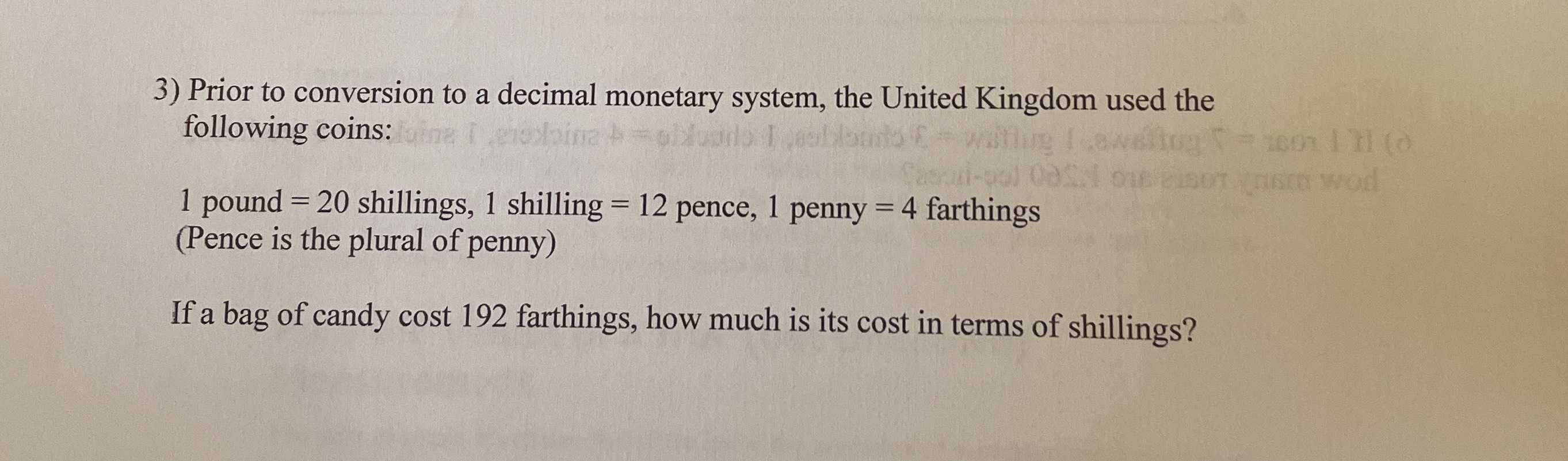### Still have math questions?

Arithmetic
Question3) Prior to conversion to a decimal monetary system, the United Kingdom used the following coins:

$$1$$ pound $$= 20$$ shillings, $$1$$ shilling $$= 12$$ pence, $$1$$ penny $$= 4$$ farthings (Pence is the plural of penny) If a bag of candy cost $$192$$ farthings, how much is its cost in terms of shillings?